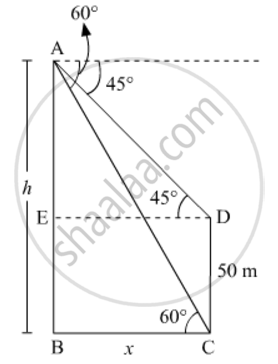# The angles of depression of the top and bottom of a 50 m high building from the top of a tower are 45° and 60° respectively. Find the height of the tower and the horizontal distance between the tower and the building - Mathematics

The angles of depression of the top and bottom of a 50 m high building from the top of a tower are 45° and 60° respectively. Find the height of the tower and the horizontal distance between the tower and the building (use sqrt3=1.73)

#### SolutionLet the height of the tower AB be h m and the horizontal distance between the tower and the building BC be x m.

So,

AE=(h50) m

In AED,

tan45^@=(AE)/(ED)

=>1=(h-50)/x

h50   .....(1)

In ABC,

tan^@=(AB)/(BC)

=>sqrt3=h/x

=>x=sqrt3=h

Using (1) and (2), we get

x=sqrt3x-50

=>x=(sqrt3-1)=50

=>x=(50(sqrt3+1))/2=25xx2.73=68.25m

Substituting the value of x in (1), we get

68.25 50

⇒ 68.25 50

h=118.25 m

Hence, the height of tower is 118.25 m and the horizontal distance between the tower and the building is 68.25 m.

Concept: Heights and Distances
Is there an error in this question or solution?